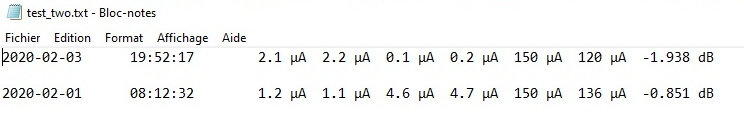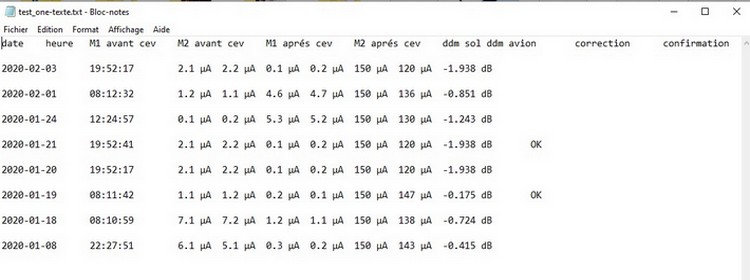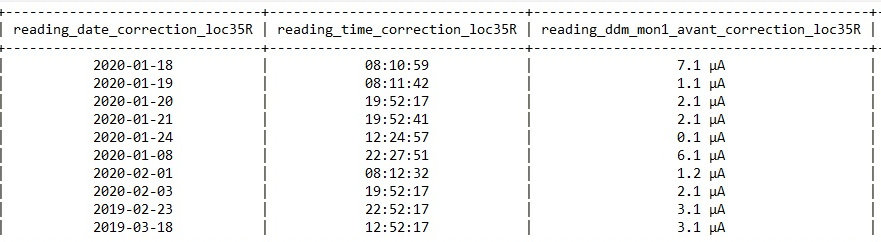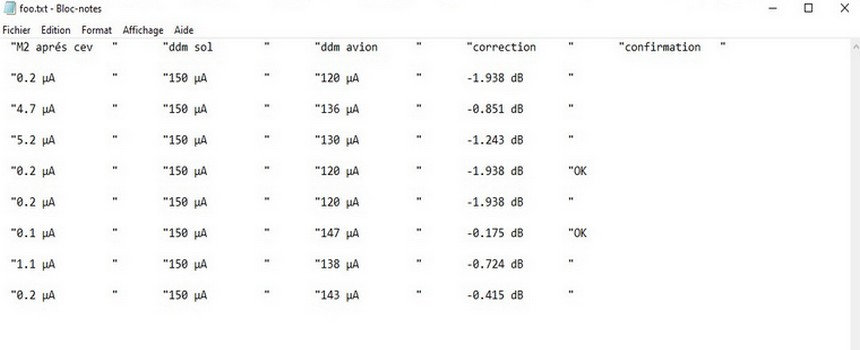# Python Forum

Full Version: saving text file by FileDialog option
You're currently viewing a stripped down version of our content. View the full version with proper formatting.
Pages: 1 2
Hi, this is the code that i was able to realize by following steps seen on one of the forums :
```def create_file_from_tableWidget(self):
path,_ = QFileDialog.getSaveFileName(
self, 'Save File', '', 'CSV(*.txt)')
if path:
with open(path, 'w',encoding="utf-8")  as stream:
writer = csv.writer(stream, delimiter='\t')
for row in range(self.tableWidget_search.rowCount()):
rowdata = []
for column in range(self.tableWidget_search.columnCount()):
item = self.tableWidget_search.item(row, column)
if item is not None:
rowdata.append(
item.text())
else:
rowdata.append('')
writer.writerow(rowdata)
```
the result is like at on the following picture :what is missing in saving the contents of the tabewidget in the text file is the header of the tabewidgetmy wish is how to add the header of the tablewidgte at the top of the text file

```    def create_file_from_tableWidget(self):
for column in range(self.tableWidget_search.columnCount()):

path,_ = QFileDialog.getSaveFileName(
self, 'Save File', 'test.csv', 'CSV(*.txt)')
if path:
with open(path, 'w',encoding="utf-8")  as stream:
writer = csv.writer(stream, delimiter='\t')
for row in range(self.tableWidget_search.rowCount()):
rowdata = []
for column in range(self.tableWidget_search.columnCount()):
item = self.tableWidget_search.item(row, column)
if item is not None:
rowdata.append(item.text())
else:
rowdata.append('')
writer.writerow(rowdata)
```
thank you for information,
I modified the line 8 to have text file as output :
`self, 'Save File', '', 'CSV(*.txt)')`
but I have a problem relating to the positioning of the columns which are badly alignedis there a solution to this problem
thank you
No, if you're using a texteditor to show it
but with prettytable librairy I save the text file with correct positioning of the columns
with this code :

```from prettytable import from_db_cursor

def create_file_from_database(self):

conn = sqlite3.connect ('database.db')
curseur=conn.cursor()
c=curseur.execute("SELECT * FROM table")

mytable = from_db_cursor(c)
table_txt = mytable.get_string()

with open("textFile.txt", "w",encoding="utf-8") as file_loc35R:
file_loc35R.write(table_txt)

conn.commit()
curseur.close()
conn.close()  ```
ther is the picture of text file with nice displaySo simply emulate that by implementing a print routine that handles the output in a pretty fashion

All you need to do is figure out the widths of each column and then add the necessary spacing to maintain it
I modify the code by adding some lines:
```def create_file_from_tablewidget(self):

for column in range(self.tableWidget.columnCount()):

path,_ = QFileDialog.getSaveFileName(
self, 'Save File', '', 'CSV(*.txt)')
if path:
with open(path, 'w',encoding="utf-8")  as stream:
writer = csv.writer(stream, delimiter='\t')
for row in range(self.tableWidget.rowCount()):
rowdata = []
for column in range(self.tableWidget.columnCount()):
item = self.tableWidget.item(row, column)
if item is not None:
rowdata.append(item.text()+"\t" * (longueur - len(item.text())))
else:
rowdata.append('')
writer.writerow(rowdata)
```
the result is like that :

the picture of the beginner of text file :the picture of the end of text file :it is better than at the beginning, but it is a bit ugly: the confirmation ("OK") is not well aligned in the confirmation column
And that is because your not owning the output -- create a function that represents a line -- within this function map out the elements of that line such as the following (note the following is pseudo code you will need to translate that into working code):
```#These would be the Headers
ColIdx = 0.1
For Each ItmId in HdrItem:
RowElements[ColIdx] = HdrItem[ItmId]
ColIdx += 0.1

MaxCol = ColIdx -= 0.1
ColIdx = 0.1
RowIdx = 1
For Each RowId in DataFeed:
RowElements[(RowId+ColIdx)] = DataFeed[RowId]
ColIdx += 0.1
if ColIdx > MaxCol:
RowIdx += 1
ColIdx = 0.1

# This gets the maximum width of each column
for each RowCol in RowElements:
Col = ConvertRowColtoCol(RowCol)
if ColWidth[Col] < len(RowElements[RowCol]):
ColWidth[Col] = len(RowElements[RowCol])

# Now using these 2 Dictionaries you build a string output from them such that
RowIdx = 0
for each RowCol in RowElements:
Col = ConvertRowColtoCol(RowCol)
ColWid = ColWidth[Col]
Row += CenterCol(RowElements[RowCol],ColWid)
if Col == MaxCols:
RowOut[RowIdx] = Row
Row = ''
RowIdx += 1

for each Row in RowOut:
OutputRowToFile(Row)```
thanks for the code , but I don't understand anything
Its pseudo code -- which means it is just used to give you an idea of how to do it.

That being said what part of it are you not understanding or better yet instead of "I don't understand anything" how about you ask specific questions and I will answer those questions -- I highly doubt you do not understand this `ColIdx = 0.1` so outline a question about what it is you are struggling with so it can be explained.

However, if all you are wanting is someone to give you the functioning code to solve your problem so that you do not have to learn then say so and I will not bother wasting your's or my time.
Pages: 1 2